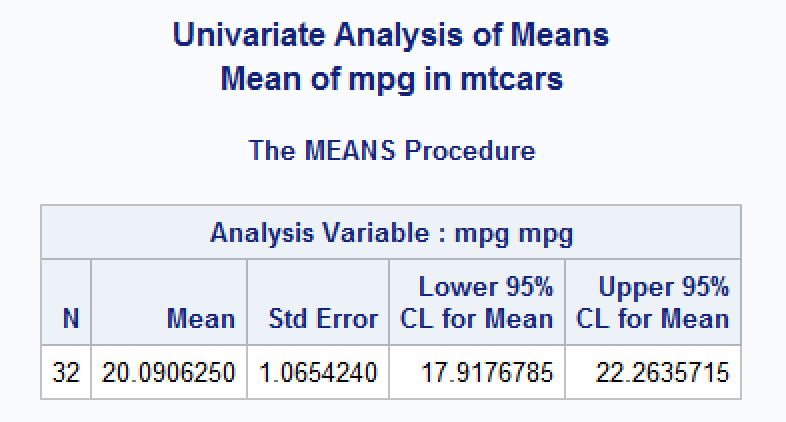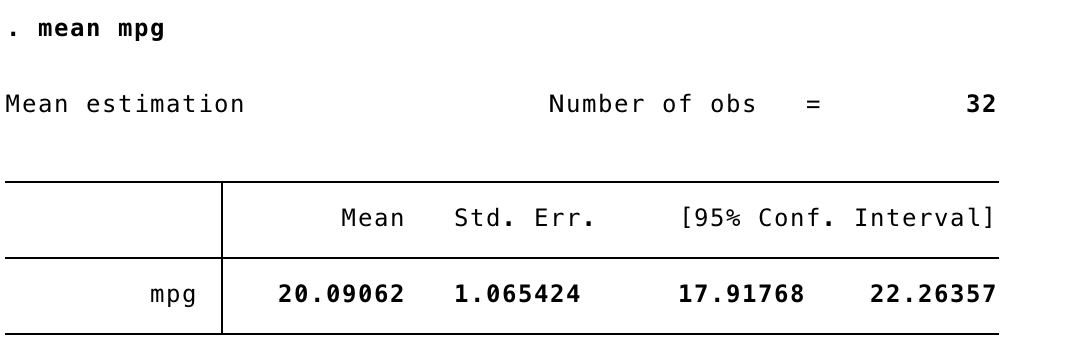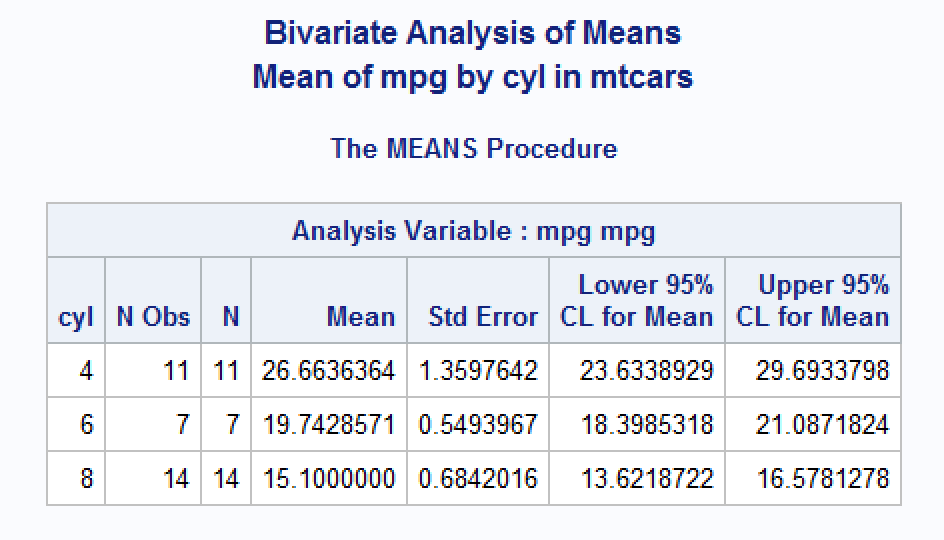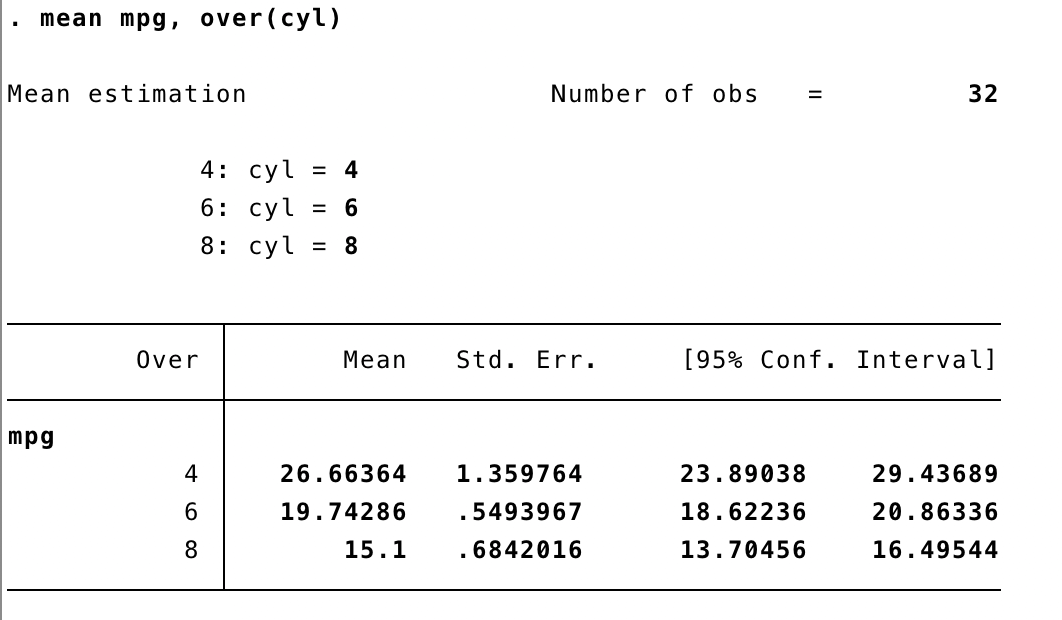# Using meantables

#### Created: 2020-07-20 Updated: 2020-07-29

library(dplyr)
#>
#> Attaching package: 'dplyr'
#> The following objects are masked from 'package:stats':
#>
#>     filter, lag
#> The following objects are masked from 'package:base':
#>
#>     intersect, setdiff, setequal, union
library(meantables)

Univariate means and 95% confidence intervals

Bivariate means and 95% confidence intervals

# Univariate means and 95% confidence intervals

In this example, we will calculate the overall mean and 95% confidence interval for the variable mpg in the mtcars data set.

By default, only the n, mean, and 95% confidence interval for the mean are returned. Additionally, the values of all the returned statistics are rounded to the hundredths place. These are the numerical summaries of the data that I am most frequently interested in. Additionally, I rarely need the precision of the estimates to be any greater than the hundredths place.

The confidence intervals are calculated as:

${\bar{x} \pm t_{(1-\alpha / 2, n-1)}} \frac{s}{\sqrt{n}}$

This matches the method used by SAS: http://support.sas.com/documentation/cdl/en/proc/65145/HTML/default/viewer.htm#p0klmrp4k89pz0n1p72t0clpavyx.htm

mtcars %>%
mean_table(mpg)
#> # A tibble: 1 x 8
#>   response_var     n  mean   sem   lcl   ucl   min   max
#>   <chr>        <int> <dbl> <dbl> <dbl> <dbl> <dbl> <dbl>
#> 1 mpg             32  20.1  1.07  17.9  22.3  10.4  33.9

By adjusting the t_prob parameter, it is possible to change the width of the confidence intervals. The example below returns a 99% confidence interval.

The value for t_prob is calculated as 1 - alpha / 2.

alpha <- 1 - .99
t <- 1 - alpha / 2

mtcars %>%
mean_table(mpg, t_prob = t)
#> # A tibble: 1 x 8
#>   response_var     n  mean   sem   lcl   ucl   min   max
#>   <chr>        <int> <dbl> <dbl> <dbl> <dbl> <dbl> <dbl>
#> 1 mpg             32  20.1  1.07  17.2  23.0  10.4  33.9

With the output = "all" option, mean_table also returns the number of missing values, the critical value from student’s t distribution with degrees of freedom n - 1, and the standard error of the mean.

We can also control the precision of the statistics using the digits parameter.

mtcars %>%
mean_table(mpg, output = "all", digits = 5)
#> # A tibble: 1 x 10
#>   response_var n_miss     n  mean t_crit   sem   lcl   ucl   min   max
#>   <chr>         <int> <int> <dbl>  <dbl> <dbl> <dbl> <dbl> <dbl> <dbl>
#> 1 mpg               0    32  20.1   2.04  1.07  17.9  22.3  10.4  33.9

This output matches the results obtained from SAS proc means and the Stata mean command (shown below).Finally, the object returned by mean_table is given the class mean_table when the data frame passed to the .data argument is an ungrouped tibble.

top

# Bivariate means and 95% confidence intervals

The methods used to calculate bivariate means and confidence are identical to those used to calculate univariate means and confidence intervals. Additionally, all of the options shown above work identically for bivariate analysis. In order to estimate bivariate (subgroup) means and confidence intervals over levels of a categorical variable, the .data argument to mean_table should be a grouped tibble created with dplyr::group_by. Everything else should “just work.”

The object returned by mean_table is given the class mean_table_grouped when the data frame passed to the .data argument is a grouped tibble (i.e., grouped_df).

mtcars %>%
group_by(cyl) %>%
mean_table(mpg, output = "all", digits = 5)
#> # A tibble: 3 x 12
#>   response_var group_var group_cat n_miss     n  mean t_crit   sem   lcl   ucl
#>   <chr>        <chr>         <dbl>  <int> <int> <dbl>  <dbl> <dbl> <dbl> <dbl>
#> 1 mpg          cyl               4      0    11  26.7   2.23 1.36   23.6  29.7
#> 2 mpg          cyl               6      0     7  19.7   2.45 0.549  18.4  21.1
#> 3 mpg          cyl               8      0    14  15.1   2.16 0.684  13.6  16.6
#> # … with 2 more variables: min <dbl>, max <dbl>

For comparison, here is the output from SAS proc means and the Stata mean command.The method used by Stata to calculate subpopulation means and confidence intervals is available here: https://www.stata.com/manuals13/rmean.pdf

top